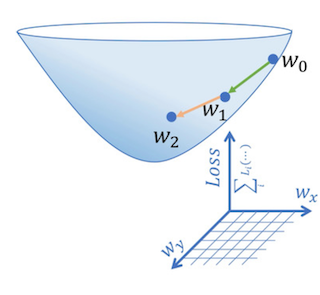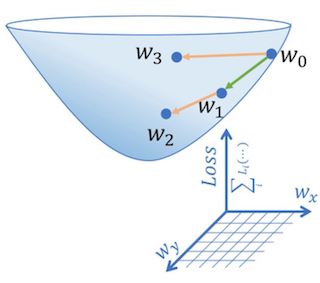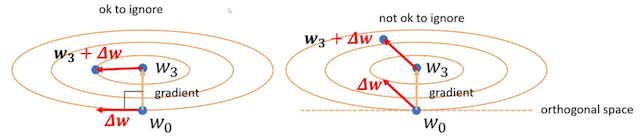Data-parallel distributed training of deep neural networks partitions the training dataset into N subsets with each of the N compute nodes training a subset of the data. By setting the minibatch size equal to that in the single node training, we essentially increase the effective batch size by N times and therefore improve the training throughput.

Increasing the effective batch size, however, will have negative impact on training accuracy discussed in . The solution proposed in  was to scale the learning rate proportional to the total batch size. However, it will still reach an upper bound where training cannot converge even with learning rate scaling.

Paper  titled Scaling Distributed Training with Adaptive Summation gave a very intuitive explanation on a key component that caused the accuracy divergence between single-node training and distributed training. It then proposed an enhanced algorithm to sum up the gradients based on the descending direction and showed that it can help reduce the convergence time by 30%.

### Motivation ExampleFig. 1. SGD on two data batches sequentially.Fig. 2. SGD on two data batches in distributed training.

Fig. 1. shows an example of performing gradient descent on two batches of data on a single node sequentially. Starting at w0, the loss moves to w1 after the performing gradient descent on the first step; and moves from w1 to w2 after the second step. Fig. 2. shows an example of performing gradient descent with the same batch size but on two training nodes concurrently. In this case, the gradient descent on the two training nodes both start at w0. After one step, the loss in one node ended up at w1 and the loss in the other node ended up at w3. In distributed training, the arithmetic average of gradients from both nodes are applied to the original weight w0 and the resulting weight w2' are used to update weights on both nodes. In most cases, this updated weight w2' will be different from w2 and the authors in  argue this is the source of accuracy discrepancy between single-node training and distributed training.

The paper went on to study the cases when w2 and w2' differ the most. They observe in Fig. 3. below that when w1 and w3 are two orthogonal vectors, the difference between w2 and w2' can be ignored; but when they are along the same direction, the difference between w2 and w2' are very significant.Fig. 3. The different cases of gradient vector summation.

Based on this observation, the paper suggests that instead of using a simple allreduce operation to aggregate gradients we should consider the correlation between gradient vectors. The authors proposed a method called AdaSum to add the gradient vectors when they are are orthogonal and perform arithmetic average when the direction of gradient vectors are in parallel. For more details about this algorithm, please refer to the original paper .

### Results

The authors in  showed that with AdaSum operation the BERT Large training can converge 30% faster than regular Allreduce. The operator has around 10~15% latency overhead compared with NCCL allreduce, but it is often an insignificant portion of the total step time. The AdaSum operator is implemented and ready to use in the Horovod distributed training library.

 Priya Goyal et al., Accurate, Large Minibatch SGD: Training ImageNet in 1 Hour (2017), CVPR 2017.

 Saeed Maleki et al., Scaling Distributed Training with Adaptive Summation (2021), MLSys 2021.Understanding AdaSum: Adaptive Summation Method for Scaling Distributed Training was originally published in Towards Data Science on Medium, where people are continuing the conversation by highlighting and responding to this story.